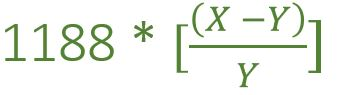Open in App
Not now

# Program to find the Speed of train as per speed of sound

• Last Updated : 28 May, 2022

Two guns were fired from same place at an interval of ‘X’ minutes. A person who is approaching the shooting place by train hears the shooting sound at an interval of ‘Y’ minutes, The task is to find the ‘S’ i.e speed of the train.
Note: Speed of the sound in air is 330m/s in a fixed value.

Examples:

Input: X = 14 min, Y = 13.5 min
Output: S = 44 km/hr

Input: X = 8 min, Y = 7.2 min
Output: S = 132 km/hr

Approach: Take the first example,

• Distance covered by the train in Y minutes
= Distance covered by the sound in (X – Y) minutes
= 330 * (X – Y) * 60 minutes (Distance = speed of sound * time)
• Speed of the train
= (330 * (X – Y) * 60 ) / Y (Speed = distance / time)
= (330 * 60 * (X – Y)) / 1000 * (60 / y)
= 1188 [ (X – Y) / Y] km/hr
• Now, According to 1st example
Time difference i.e (X – Y) = 14.00 – 13.30 = 30sec
Speed of train = 1188 * [ (14 – 13.5) / 13.5 ] = 44km/hr
• This can be calculated by using the below formula:Below is the implementation of the above approach.

## C++

 `// C++ implementation of the approach` `#include ``using` `namespace` `std;` `// Function to find the``// Speed of train``float` `speedOfTrain(``float` `X, ``float` `Y)``{` `    ``float` `Speed = 0;` `    ``Speed = 1188 * ((X - Y) / Y);` `    ``return` `Speed;``}` `// Driver code``int` `main()``{``    ``float` `X = 8, Y = 7.2;` `    ``// calling Function``    ``cout << speedOfTrain(X, Y)``         ``<< ``" km/hr"``;` `    ``return` `0;``}`

## Java

 `// Java implementation of the approach``class` `GFG``{` `    ``// Function to find the``    ``// Speed of train``    ``static` `int` `speedOfTrain(``float` `X, ``float` `Y)``    ``{``        ``float` `Speed;` `        ``Speed = ``1188` `* ((X - Y) / Y);` `        ``return` `(``int``)Speed;``    ``}` `    ``// Driver code``    ``public` `static` `void` `main(String[] args)``    ``{``        ``float` `X = 8f, Y = ``7``.2f;` `        ``// calling Function``        ``int` `result = (speedOfTrain(X, Y));``        ``System.out.println(result + ``" km/hr"``);``    ``}``}` `// This code is contributed by PrinciRaj1992`

## Python3

 `# Python3 implementation of the approach``from` `math ``import` `ceil` `# Function to find the``# Speed of train``def` `speedOfTrain(X, Y):``    ``Speed ``=` `0` `    ``Speed ``=` `1188` `*` `((X ``-` `Y) ``/` `Y)` `    ``return` `Speed` `# Driver code``if` `__name__ ``=``=` `'__main__'``:``    ``X ``=` `8``    ``Y ``=` `7.2` `    ``# calling Function``    ``print``(ceil(speedOfTrain(X, Y)),``                   ``end ``=` `" km/hr"``)` `# This code is contributed by``# Surendra_Gangwar`

## C#

 `// C# implementation of the approach``using` `System;` `class` `GFG``{` `    ``// Function to find the``    ``// Speed of train``    ``static` `int` `speedOfTrain(``float` `X, ``float` `Y)``    ``{``        ``float` `Speed;` `        ``Speed = 1188 * ((X - Y) / Y);` `        ``return` `(``int``)Speed;``    ``}` `    ``// Driver code``    ``public` `static` `void` `Main()``    ``{``        ``float` `X = 8f, Y = 7.2f;` `        ``// calling Function``        ``int` `result = (speedOfTrain(X, Y));``        ``Console.Write(result + ``" km/hr"``);``    ``}``}` `// This code is contributed by anuj_67..`

## Javascript

 ``

Output:

`132 km/hr`

Time Complexity: O(1)

Auxiliary Space: O(1)

My Personal Notes arrow_drop_up